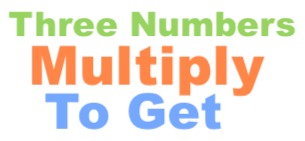What three numbers multiply to get 64?What three numbers multiply to get 64? In other words, you want to know all the combinations of 3 numbers that can be multiplied to get 64. You want to know what all the question marks in the equation below can be in order for the equation to be true:

? × ? × ? = 64

First, note that all numbers must be 64 or less, which means that there are 64 × 64 × 64 possible solutions. Thus, to get the answer to "What three numbers multiply to get 64?" we looked at 262,144 different combinations to see which ones equal 64.

When we looked at all those combinations, we found 28 different combinations of 3 numbers that equal 64 when multiplied together. Here are all 3 number combinations that you can multiply to get 64:

64 × 1 × 1 = 64
32 × 2 × 1 = 64
32 × 1 × 2 = 64
16 × 4 × 1 = 64
16 × 2 × 2 = 64
16 × 1 × 4 = 64
8 × 8 × 1 = 64
8 × 4 × 2 = 64
8 × 2 × 4 = 64
8 × 1 × 8 = 64
4 × 16 × 1 = 64
4 × 8 × 2 = 64
4 × 4 × 4 = 64
4 × 2 × 8 = 64
4 × 1 × 16 = 64
2 × 32 × 1 = 64
2 × 16 × 2 = 64
2 × 8 × 4 = 64
2 × 4 × 8 = 64
2 × 2 × 16 = 64
2 × 1 × 32 = 64
1 × 64 × 1 = 64
1 × 32 × 2 = 64
1 × 16 × 4 = 64
1 × 8 × 8 = 64
1 × 4 × 16 = 64
1 × 2 × 32 = 64
1 × 1 × 64 = 64

Three Numbers Multiply to Get Calculator
Do you need a different combination of three numbers that when multiplied equal a number other than 64? No problem! Please enter your number here.

What three numbers multiply to get 65?
Here is the next problem on our list that we have explained and answered for your convenience.